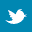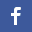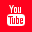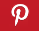Completely Solved C, C++ Programs Assignment.Quick Search Database Videos Tutorials Ebooks Forums FAQ Aboutus Household Industrial Manufacturing Service Shopping Transportation### C program to implement Bisection method to solve an Algebraic or Transcendal equation.

 Filed Under: C Assignments

Program Statement :
Write a C program to implement Bisection method to solve an Algebraic or Transcendal equation.

Theory :
The method of bisection is a root-finding algorithm which repeatedly bisects an interval then selects a subinterval in which a root must lie for further processing. It is the most simplest iterative and robust method, but it is also relatively slow. It is also known as half-interval or Bolzano method. This method is based on the following theorem on the change of sign.
Theorem : If f(a) and f(b) are of the same sign, then there are an odd number (at least one) of real roots of the equation f(x) = 0 between a and b.
In this method, we first find out a sufficiently small interval [a0, b0] containing the required root by graphical or tabulation method. Then f(a0)f(b0) < 0 and f'(x) has the same sign in [a0, b0] and so f(x) is strictly monotonic in [a0, b0]. To generate the sequence {xn} of iterates, we put x0 = a0 or b0 and x1 = ½ (a0 + b0) and find f(x1). If f(a0) and f(x1) are of opposite signs, then set a1 = a0, b1 = x1 so that [a1, b1] = [a0, x1]. On the other hand, if f(x1) and f(b0) are of opposite signs then put a1 = x1, b1 = b0, i.e. [a1, b1] = [x1, b0]. Thus we see that [a1, b1] contains the root ∝ in either case. Next set x2 = ½ (a1 + b1) and repeat the above process till we obtain xn+1 = ½ (an + bn) with desired accuracy with xn → ∝ as n → ∞.

A few steps of the bisection method applied over the starting range [a1, b1]. The bigger dot is the root of the function.

Algorithm :
Step1 : Start the program.
Step2 : Define the function f(x).
Step3 : Select he interval (a, b) in which the root lies.
Step4 : Calculate y1 = f(a), y2 = f(b).
Step5 : If y1y2 < 0, then goto step 6 else step 10. Step6 : Calculate x = (a+b)/2, y = f(x). Step7 : If y1y2 < 0, set b = x, otherwise set a = x. Step8 : If |a-b| < ℇ, ℇ being the prescribed accuracy, then go to step 9 else step 6. Step9 : Print the value of x. Step10 : Stop the program.

Program listing :
/* C program to find a real root of a given equation using Bisection method */
#include<stdio.h>
#include<math.h>
#define e 0.000001/*e is the prescribed accuracy*/
main()
{
double g1,g2,g,v,v1,v2,prev;
int found=0,converged=0,i=0;
double f(double);
printf("We apply Bisection method to find a real root of the non-linear equation f(x) = 0, where f(x) = x^(2.7182818)-3cosx+1n");
while(found==0)/*This loop will continue until a range is found in between which a real root lies*/
{
printf("nEnter the first guess : ");
scanf("%lf",&g1);
v1=f(g1);
printf("nEnter the second guess : ");
scanf("%lf",&g2);
v2=f(g2);
if(v1*v2>0)
{
found=0;
g1++;
printf("nRoot does not lie in [%lf,%lf].n",g1-1,g2);
printf("nn..Enter two new guesses..nn");/*Previous two guesses are inappropriate*/
}
else
found=1;
}
printf("nThere is a real root which lies in [%lf,%lf].n",g1,g2);
while(converged==0)/*This loop will continue until a real root is found*/
{
printf("nnIteration = %dnn",i);
printf("a[%d](-ve)tb[%d](+ve)tbbx[%d]=(a[%d]+b[%d])/2tf(x[%d])n",i,i,i+1,i,i,i+1);
printf("%lft",g1);
printf("%lft",g2);
g=(g1+g2)/2;
v=f(g);
printf("%lft",g);
printf("t%lf",v);
if(v<0)
g1=g;
else
g2=g;
if(fabs(prev-v)<e)
converged=1;
else
prev=v;
i=i+1;
}
printf("nnThe approximate value of the root is : %lfn",g);
}
/*This function returns values of f(x)*/
double f(double x)
{
return pow(2.7182818,x)-3*cos(x)+1;

}

Output :
We apply Bisection method to find a real root of the non-linear equation f(x) = 0, where f(x) = x^(2.7182818)-3cosx+1

Enter the first guess : 1
Enter the second guess : 2

Root does not lie in [1.000000,2.000000].

..Enter two new guesses..

Enter the first guess : 0
Enter the second guess : 1

There is a real root which lies in [0.000000,1.000000].

Iteration = 0

a(-ve) b(+ve) x=(a+b)/2 f(x)
0.000000 1.000000 0.500000 0.015974

Iteration = 1

a(-ve) b(+ve) x=(a+b)/2 f(x)
0.000000 0.500000 0.250000 -0.622712

Iteration = 2

a(-ve) b(+ve) x=(a+b)/2 f(x)
0.250000 0.500000 0.375000 -0.336531

Iteration = 3

a(-ve) b(+ve) x=(a+b)/2 f(x)
0.375000 0.500000 0.437500 -0.168611

Iteration = 4

a(-ve) b(+ve) x=(a+b)/2 f(x)
0.437500 0.500000 0.468750 -0.078406

Iteration = 5

a(-ve) b(+ve) x=(a+b)/2 f(x)
0.468750 0.500000 0.484375 -0.031738

Iteration = 6

a(-ve) b(+ve) x=(a+b)/2 f(x)
0.484375 0.500000 0.492188 -0.008013

Iteration = 7

a(-ve) b(+ve) x=(a+b)/2 f(x)
0.492188 0.500000 0.496094 0.003948

Iteration = 8

a(-ve) b(+ve) x=(a+b)/2 f(x)
0.492188 0.496094 0.494141 -0.002041

Iteration = 9

a(-ve) b(+ve) x=(a+b)/2 f(x)
0.494141 0.496094 0.495117 0.000951

Iteration = 10

a(-ve) b(+ve) x=(a+b)/2 f(x)
0.494141 0.495117 0.494629 -0.000545

Iteration = 11

a(-ve) b(+ve) x=(a+b)/2 f(x)
0.494629 0.495117 0.494873 0.000203

Iteration = 12

a(-ve) b(+ve) x=(a+b)/2 f(x)
0.494629 0.494873 0.494751 -0.000171

Iteration = 13

a(-ve) b(+ve) x=(a+b)/2 f(x)
0.494751 0.494873 0.494812 0.000016

Iteration = 14

a(-ve) b(+ve) x=(a+b)/2 f(x)
0.494751 0.494812 0.494781 -0.000078

Iteration = 15

a(-ve) b(+ve) x=(a+b)/2 f(x)
0.494781 0.494812 0.494797 -0.000031

Iteration = 16

a(-ve) b(+ve) x=(a+b)/2 f(x)
0.494797 0.494812 0.494804 -0.000008

Iteration = 17

a(-ve) b(+ve) x=(a+b)/2 f(x)
0.494804 0.494812 0.494808 0.000004

Iteration = 18

a(-ve) b(+ve) x=(a+b)/2 f(x)
0.494804 0.494808 0.494806 -0.000002

Iteration = 19

a(-ve) b(+ve) x=(a+b)/2 f(x)
0.494806 0.494808 0.494807 0.000001

Iteration = 20

a(-ve) b(+ve) x=(a+b)/2 f(x)
0.494806 0.494807 0.494807 -0.000000

Iteration = 21

a(-ve) b(+ve) x=(a+b)/2 f(x)
0.494807 0.494807 0.494807 0.000001

The approximate value of the root is : 0.494807

Discussions :
 If f has several simple roots in the interval [a, b], then the bisection method will find one of them.
 The expression f(left) * f(midpoint) is very likely to underflow to 0 since both arguments are approaching a zero of f. To avoid this possibility, the two function values should be tested separately rather than multiplied.
 If ℇ is too small, the value of |(right – left)| might never become as small as 2*ℇ, as left and right can get stuck at adjacent non-equal floating-point values.
 The order of convergence of bisection method is 1, i.e., the convergence is linear.
 Advantage of bisection method is that it is very simple, as at any stage of iteration the approximate value of the desired root of the equation f(x) = 0 does not depend on the values f(xn) but on their signs only.
 Also this method is unconditionally and surely convergent if f(a) and f(b) have different signs.
 Disadvantage of this method is that it is very slow and requires large number of iteration to obtain moderately accurate results and hence it is laborious.Back to main directory:Software Practical